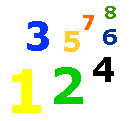# Multiplying Fractions

Multiplying Fractions Lesson & Worksheet - My Schoolhouse - Online Learning

Multiplying fractions.  Look at the examples below.

 7 X 4 = 28 or 7 8 5 40 10

 5 X 3 = 15 or 1 6 5 30 2

 3 X 2 = 6 or 3 8 5 40 20

 7 X 4 = 28 or 7 8 5 40 10

Solve the equations below.

 5 X 4 = 20 or 1 8 5

 2 X 3 = 6 or 2 3 7

 3 X 2 = 6 or 2 5 9

 7 X 2 = 14 or 7 10 3

 2 X 9 = or 3 10

 1 X 2 = or 12 3

 5 X 4 = or 9 5

 4 X 7 = or 5 10

 1 X 8 = or 2 15

 2 X 1 = or 3 8

 5 X 9 = or 7 10

 8 X 1 = or 17 2

 6 X 4 = or 7 10

 2 X 6 = or 3 7

 1 X 9 = or 12 1

 1 X 9 = or 3 10

 7 X 2 = or 12 3

 1 X 9 = or 2 15

 2 X 9 = or 3 18

 5 X 6 = or 14 7